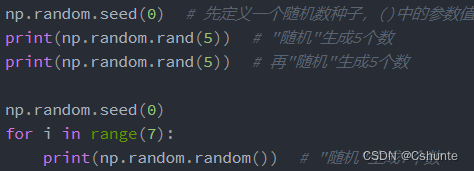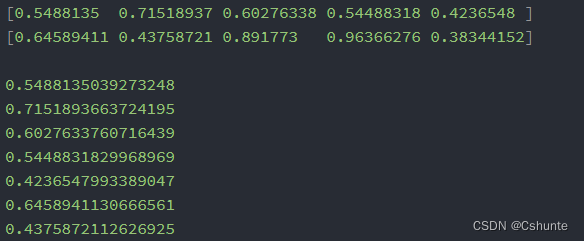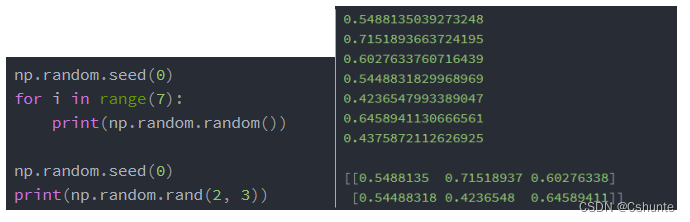• python随机数
2022-04-19 09:19:34
import xlwt
import random
def write_excel_data():
workbook = xlwt.Workbook(encoding = 'ascii')
for i in range(6114):              # 生成6114条数据
worksheet.write(i, 1, random.randint(20 ,180))  # 不带样式的写入（行，列，数据）

workbook.save('22.xls')  # 保存文件

if __name__ == '__main__':
write_excel_data()

更多相关内容
• 本文实例讲述了Python随机数用法。分享给大家供大家参考，具体如下： 1. random.seed(int) 给随机数对象一个种子值，用于产生随机序列。 对于同一个种子值的输入，之后产生的随机数序列也一样。 通常是把时间秒数等...
• python随机数In this tutorial, we are going to learn about Python Random Number. In our previous tutorial, we learned about Python math module. 在本教程中，我们将学习Python随机数。 在上一教程中，我们...

python随机数

In this tutorial, we are going to learn about Python Random Number. In our previous tutorial, we learned about Python math module.

在本教程中，我们将学习Python随机数。 在上一教程中，我们了解了Python数学模块

## Python随机数 (Python Random Number)

To work with python random number, we need to import Python’s random module at first. Python random module provides pseudo-randomness.

要使用python随机数，我们首先需要导入Python的random模块。 Python随机模块提供伪随机性。

Python random module uses Mersenne Twister as the core random generator. So, this module is completely unsuitable for cryptographic purposes for being deterministic. However, we can use Python’s random module for most of the cases because Python’s random module contains many well known random distributions.

Python随机模块使用Mersenne Twister作为核心随机生成器。 因此，该模块完全不适合用于确定性的加密目的。 但是，在大多数情况下，我们可以使用Python的随机模块，因为Python的随机模块包含许多众所周知的随机分布。

### Python随机整数 (Python Random Integer)

In this section, we will be discussing about generation integer numbers randomly. We can use randint(a,b) function to get a random integer from range a to b. Again, we can get number from a sequence by using randrange(start, stop, step) function. Let’s see an example to get python random integer.

在本节中，我们将随机讨论生成整数。 我们可以使用randint(a,b)函数来获取范围从ab的随机整数。 同样，我们可以使用randrange(start, stop, step)函数从序列中获取数字。 让我们看一个获取python随机整数的示例。

import random as rand

a = 10
b = 100
print('\na =', a, 'and b =', b)
print('printing number [', a, ', ', b, ') :', rand.randint(a,b))

start = 1
stop = 12
step = 2
print('\nsequence = [1, 3, 5, 7, 9, 11]')
print('printing one number from the sequence :', rand.randrange(start, stop, step))

For each run, the output will change. However, here given a sample output.

对于每次运行，输出都会改变。 但是，这里给出了示例输出。

### Python随机浮动 (Python Random Float)

There are several functions that returns real number or float randomly. For example, random() function returns a real number from 0 to 1 (exclusive).

有几个函数可以返回实数或随机浮动。 例如， random()函数返回一个从0到1（不包括）的实数。

Again, uniform(a, b) functions return a real number from a to b. Moreover there are some random distributions also available in Python random module. We can also get real number from those distribution.

同样， uniform(a, b)函数将实数从a返回到b。 此外，Python随机模块中还提供了一些随机分布。 我们还可以从这些分布中获得实数。

We can get random numbers from exponential distribution by using expovariate(lambd) function.

我们可以使用expovariate（lambd）函数从指数分布中获得随机数。

import random as rand

print('Random number from 0 to 1 :', rand.random())
print('Uniform Distribution [1,5] :', rand.uniform(1, 5))
print('Gaussian Distribution mu=0, sigma=1 :', rand.gauss(0, 1))
print('Exponential Distribution lambda = 1/10 :', rand.expovariate(1/10))

The values in output will vary for each execution. You will get output like this.

输出中的值对于每次执行都会有所不同。 您将获得这样的输出。

Random number from 0 to 1 : 0.5311529501408693
Uniform Distribution [1,5] : 3.8716411264052546
Gaussian Distribution mu=0, sigma=1 : 0.8779046620056893
Exponential Distribution lambda = 1/10 : 1.4637113187536595

### Python随机种子 (Python Random seed)

Python random number generation is based on the previous number, so using system time is a great way to ensure that every time our program runs, it generates different numbers.

Python随机数的生成基于先前的数字，因此使用系统时间是确保每次程序运行时都会生成不同数字的好方法。

We can use python random seed() function to set the initial value. Note that if our seed value doesn’t change in each execution, we will get same sequence of numbers. Below is a sample program to prove this theory about seed value.

我们可以使用python random seed（）函数来设置初始值。 请注意，如果我们的种子值在每次执行中都没有改变，我们将获得相同的数字序列。 下面是一个示例程序，用于证明有关种子价值的这一理论。

import random

random.seed(10)

print('1st random number = ', random.random())
print('2nd random number = ', random.random())
print('1st random int = ', random.randint(1, 100))
print('2nd random int = ', random.randint(1, 100))

# resetting the seed to 10 i.e. first value
random.seed(10)

print('3rd random number = ', random.random())
print('4th random number = ', random.random())
print('3rd random int = ', random.randint(1, 100))
print('4th random int = ', random.randint(1, 100))

Below image shows the output produced by the python random seed example program. We will get the same sequence of random numbers for each run.下图显示了python随机种子示例程序产生的输出。 对于每次运行，我们将获得相同的随机数序列。

### Python随机列表– choice（），shuffle（），sample（） (Python Random List – choice(), shuffle(), sample())

There are some functions to use randomness in a sequence. For example, using choice() function you can get a random element from a sequence.

有一些函数可以在序列中使用随机性。 例如，使用choice()函数可以从序列中获取随机元素。

Again, using shuffle() function you can shuffle the elements in a sequence.

同样，使用shuffle()函数可以将序列中的元素随机播放。

Also, using sample() function you can get x number of elements from a sequence randomly. So, let’s see the following code for random list example.

另外，使用sample()函数可以从序列中随机获取x个元素。 因此，让我们看下面的随机列表示例代码。

import random as rand

# initialize sequences
string = "inconvenience"
l = [1, 2, 3, 4, 10, 15]

# get a single element randomly
print('Single character randomly chosen :', rand.choice(string))
print('one randomly chosen number :', rand.choice(l))

# get multiple element
print('Randomly chosen 4 character from string :', rand.sample(string, 4))
print('Randomly chosen 4 length list :', rand.sample(l, 4))

# shuffle the list
rand.shuffle(l)
print('list is shuffled :', l)  # print the list

You may get output like the following.

您可能会得到类似以下的输出。

Single character randomly chosen : i
one randomly chosen number : 10
Randomly chosen 4 character from string : ['e', 'c', 'n', 'n']
Randomly chosen 4 length list : [2, 10, 3, 15]
list is shuffled : [2, 4, 15, 3, 10, 1]

So, that’s all for python random number. To know more, see their official documentation.

所以，这就是python随机数的全部。 要了解更多信息，请参阅其官方文档

python随机数

展开全文random 机器学习 java 人工智能
• 0 与 1 之间的随机浮点数N二、random.uniform(a,b)返回 a 与 b 之间的随机浮点数N三、random.randint(a,b)返回一个随机的整数N四、random.randrange([start], stop[, step])返回指定递增基数集合中的一个随机数五、...
• 之后按照固定顺序生成随机数 随机数种子对后面的结果一直有影响，在一个随机数种子后生成的随机数都会收到这个随机数种子的影响，即生成的随机数都是由这个随机数种子给的初值，按照固定顺序生成的（生成的随机数受...
np.random.seed（）

在生成随机数时，我们可以利用随机数种子，控制生成的随机数。（利用随机数种子，得到相同或者部分形同的随机数）

随机数种子，相当于给需要生成的随机数一个初值，按照这个初值，依据固定顺序生成随机数。

两例子：

一、输出结果如下：可以看到第二次生成的随机数和之前的一样

二、因此想要生成两个一样的随机矩阵，在生成矩阵之前定义随机种子就好了。

总结：

1. 随机数种子相当于给我们一个初值。之后按照固定顺序生成随机数
2. 随机数种子对后面的结果一直有影响，在一个随机数种子后生成的随机数都会收到这个随机数种子的影响，即生成的随机数都是由这个随机数种子给的初值，按照固定顺序生成的（生成的随机数受离它最近的随机数种子影响，即它之前的随机数种子）

np.random.seed()的参数：

初值由参数决定，只要每次这个参数相同，那我们的初值就是相同的，种子就是相同的。那么之后长得枝条就是相同的。即list是相同的

展开全文• 文章目录13 python 随机数random库的使用1.常用随机数函数2.扩展随机数函数3.随机数应用实例：蒙特卡罗方法求π 13 python 随机数random库的使用 1.常用随机数函数 设定随机数种子，可以保证程序再次执行的可重复性...
• 以下的文章主要是以介绍python随机数生成的代码来介绍Python随机数生成在实际操作过程中的具体应用，如果你对其的相关内容感兴趣的话，你就可以点击以下的文章。希望你会对它有所收获。 Python中的random模块用于...
• ## python随机数（random）

万次阅读 多人点赞 2022-01-10 00:50:02
python中的random.randint(a,b)用于生成一个指定范围内的整数。其中参数a是下限，参数b是上限，生成的随机数n: a <= n <= b。 下限必须小于等于上限，大于上限报错。 random.randint(1,50)#随机生成最小值为...

# 需要导入的库：

import random
import string


# 一、随机整数：

### 1、包含上下限：[a, b]

random.randint(a,b)


在python中的random.randint(a,b)用于生成一个指定范围内的整数。其中参数a是下限，参数b是上限，生成的随机数n: a <= n <= b。
下限必须小于等于上限，大于上限报错。

random.randint(1,50)#随机生成最小值为1，最大值为50的整数（可以等于上下限）
random.randint(20, 20)  #上下限一样时结果永远是20


### 2、不包含上限：[a, b）

参数a是下限，参数b是上限，生成的随机数n: a <= n < b。

random.randrange(a, b)


randint和randrange的区别：
randint 产生的随机数区间是包含左右极限的，也就是说左右都是闭区间的[1, n]，能取到1和n。
而 randrange 产生的随机数区间只包含左极限，也就是左闭右开的[1, n)，1能取到，而n取不到。
randint 产生的随机数是在指定的某个区间内的一个值，而 randrange 产生的随机数可以设定一个步长，也就是一个间隔。
randint 无法设定步长，会报错。

### 3、# 随机选取指定范围内指定基数递增集合中的随机数

从指定范围内，按指定基数递增的集合中 获取一个随机数。如：random.randrange(10, 100, 2)，结果相当于从[10, 12, 14, 16, … 96, 98]序列中获取一个随机数。random.randrange(10, 100, 2)在结果上与 random.choice(range(10, 100, 2) 等效

random.randrange([start], stop[, step])


### 随机选取0到100间的偶数：

 random.randrange(0, 101, 2)


# 二、随机浮点数

### 1、0-1之间的随机浮点数

0 <= n < 1.0

random.random() #用于生成一个0到1的随机符点数: 0 <= n < 1.0


### 2、随机浮点数：

random.uniform(a, b)


random.uniform(a, b)，用于生成一个指定范围内的随机符点数，两个参数其中一个是上限，一个是下限。如果a > b，则生成的随机数n: a <= n <= b。如果 a <b， 则 b <= n <= a。
（random.uniform()可以允许下限大于上限，不会报错，随机结果在a和b之间，可以等于上下限）

random.uniform(1, 10)  #随机生成1到10之间的浮点数，可等于1或10
random.uniform(10, 1)  #随机生成1到10之间的浮点数，可等于1或10


# 三、随机选取字符：

### 1、随机字符

random.choice(sequence)


random.choice从序列中获取一个随机元素。其函数原型为：random.choice(sequence)。参数sequence表示一个有序类型。这里要说明 一下：sequence在python不是一种特定的类型，而是泛指一系列的类型。list, tuple, 字符串都属于sequence。有关sequence可以查看python手册数据模型这一章。
示例：

print(random.choice('abcdefghijklmnopqrstuvwxyz!@#\$%^&*()'))
print(random.choice("学习Python"))
print(random.choice(["JGood", "is", "a", "handsome", "boy"]))
print(random.choice(("Tuple", "List", "Dict")))


运行结果：

u
P
boy
Tuple


### 2、多个字符中生成指定数量的随机字符：

print random.sample(‘zyxwvutsrqponmlkjihgfedcba’,5)

### 从a-z A-Z 0-9生成指定数量的随机字符：

 a_str = ''.join(random.sample(string.ascii_letters + string.digits, 5)) #生成5位随机字符，包括大小写字母和数字


join() 方法用于将序列中的元素以指定的字符连接生成一个新的字符串。

语法：str.join(sequence)

实例：
s1 = “-”
s2 = “”
seq = (“r”, “u”, “n”, “o”, “o”, “b”) # 字符串序列
print (s1.join( seq ))
print (s2.join( seq ))
以上实例输出结果如下：
r-u-n-o-o-b
runoob

### 3、多个字符中选取指定数量的字符组成新字符串：

random.sample(sequence, k)


从指定序列中随机获取指定长度的片断。sample函数不会修改原有序列

print(''.join(random.sample(['z','y','x','w','v','u','t','s','r','q','p','o','n','m','l','k','j','i','h','g','f','e','d','c','b','a'], 5)))


运行结果：

zlesv


# 四、打乱排序

random.shuffle(x[, random])


random.shuffle(x[, random])，用于将一个列表中的元素打乱。

items = [1, 2, 3, 4, 5, 6, 7, 8, 9, 0,"Python", "is", "powerful", "simple"]
print(items)
random.shuffle(items)
print(items)


运行结果：

[1, 2, 3, 4, 5, 6, 7, 8, 9, 0, 'Python', 'is', 'powerful', 'simple']
['Python', 1, 'is', 3, 2, 6, 'simple', 9, 'powerful', 4, 5, 8, 0, 7]

展开全文开发语言 后端
• 因为概率问题，所以需要测试一下python随机数分布。到底是平均（均匀）分布，还是正态（高斯）分布。 测试代码如下： #! /usr/bin/env python #coding=utf-8 # ================================= # Describe : ...
• 1.写一个5行代码的随机数 # 调用函数random库 import random #必须调用函数的random库 # 1.写一个5行随机数代码： #定义一个函数 def number(): # 输出5个随机数 number = random.sample([b for b in range(1, ...
• 解释：random.seed() 可以给随机数设置种子，使用相同的种子会生成相同的随机值 下面使用两个种子，一个0，一个1 相同 体现在随机数与种子的距离，与相同种子距离相同的随机数相同 import random random.seed(0...pycharm 自然语言处理
• title：这篇文章主要来介绍python随机数产生方法。1.random.random()import randomprint random.random() #0.834407670706这是一个简单创建的方法，但是我们平常需要参数一组随机数，并且要指定范围的来创建随机数。...
• 这篇文章主要介绍了Python随机数函数代码实例解析,文中通过示例代码介绍的非常详细，对大家的学习或者工作具有一定的参考学习价值,需要的朋友可以参考下 ''' choice(seq) 从序列的元素中随机选出一个元素 randrange...
• 因为概率问题，所以需要测试一下python随机数分布。到底是平均(均匀)分布，还是正态(高斯)分布。测试代码如下：#! /usr/bin/env python#coding=utf-8# =================================# Describe : 测试random...
• random.randomrandom.random()用于生成一个0到1的随机符点数: 0 b，则生成的随机数n: b
• 主要为大家详细介绍了python随机数分布random的测试，具有一定的参考价值，感兴趣的小伙伴们可以参考一下
• 1、random 库中的常用随机数生成函数 2、一些用法举例 3、随机数种子 seed(a) 的理解与使用 random 库的主要目的就是生成随机数。 1、random 库中的常用随机数生成函数 from random import * 1、seed(a=None)...random
• python随机数的产生及函数定义关键参数详解python随机数的产生学习Python的人都知道，在Python中产生随机函数的模块是random，下面是小编为大家整理的一些random模块中的常见函数的用法。当然要产生随机函数，首先是...
• 随机数可以用于数学，游戏，安全等领域中，还经常被嵌入到算法中，用以提高算法效率，并提高程序的安全性。 1、choice()函数 语法：random.choice(seq) seq—列表、元组、字符串。 返回一个列表，元组或字符串的随机...random
• Python随机数判断 import random answer = random.randint(1,100) counter = 0 while True: counter+=1 number = int(input('请输入')) if number < answer: print("小了，请输入大一点") elif number >...
• 本文给大家分享的是Python随机数random模块的几个常用的方法，非常的简单，小伙伴们喜欢的话，后续继续深入探讨
• ## python随机数种子

千次阅读 2021-01-13 20:05:44
设置随机数种子 >>> numpy.random.seed(42) #42可替换为任意整数 >>> numpy.random.randint(10) 6 >>> numpy.random.randint(10) 3 >>> numpy.random.randint(10) 7 >>&...
• 下面小编就为大家带来一篇python 随机数使用方法,推导以及字符串,双色球小程序实例。小编觉得挺不错的，现在就分享给大家，也给大家做个参考。一起跟随小编过来看看吧
• 生成随机数主要用random.randrange()或者random.randint()这两个函数 import random goal = random.randint(1,100) guess = int(input('please input a number from 1 to 100:')) while guess != goal: if guess &......